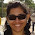Latest Articles

# Calculate Division with Remainder using Vedic Maths for 9, 99,999, 98 etc

Info Post
The beauty of maths is that there are many different ways to solve a single problem. You can use patterns, numbers and even simple tricks. We have already seen how to derive answers for any numbers divided by 9 in the "Beauty of number 9" blog post. And today we are extending this further to find answers for numbers divided by 99,98 etc.

### How to divide the number 141215 by 99?

We see that 99 has two digit numbers, so
• Rule 1: Split the number into pairs as 14 | 12 | 15 and note that the last pair will be the part of our remainder.
• Rule 2: Put the first LHS pair down, next add the LHS pair to the consecutive pairs to get the next digits and do the same until you finish adding all the pairsVedic Maths Division by 99

### How to divide the number 141215 by 99?Vedic Maths Division by 99
You might have seen how easy is to divide any even pairs of numbers by 99. Lets extend this further and see what happens when we divide the same numbers 999.

### How to divide any numbers by 999?

Again having even pairs and with 999 being three digit number, lets pair the dividend in threes.Vedic Maths Division by 999
The above examples work because the divisor is close to their base 100 ,1000 etc. And it work under the vedic maths principle of Nikhilam Navatashcaramam Dashatah division.

The Nikhilam sutra states “all from 9 and the last from 10” which means you are applying the Nikhilam formula on the divisor to get its complement. For 99,999 , our complement is 1 which when multiplied is just the same. But for divisor 98, the complement is 2 which get multiplied as we move to the next digits. For example,Vedic Mathematics Division by 98
From the above image,
Step 1- Bring 14 down as answer
Step 2- Next, double 14 ( using complement 2) and add the answer to the 12
Step 3- With the answer got from step 2, double it to add to 15. The answer got in this step is our remainder.

If you have learnt the above trick, you now can solve any numbers divisible by 9, 99, 999 and so on. And before you could ask, what if I have odd pairs of numbers? In this case, start by splitting the digits as pairs from the RHS towards the LHS. Example,Vedic Maths Division by 99
If you still have any questions, kindly ask the same through the comment below. I will answer them as soon as possible.

Share:

1.Good one Uma....very informative post, you have such a passion for Vedic maths :)

2.Wow....great information, learned a new thing...thanks for sharing

3.That was interesting

4.Very Informative! Quite helpful for the kids. Thanks for sharing!

5.i got quantitative aptitude lesson :d

6.Wow! Very interesting Uma! I always wished to learn vedic maths. You post has given a chance learn something. Thank you! I have a doubt, what to do if there are more than 6digits?

Regards,
Sindhu
Tantu
The Arts & Me

7.Cool Uma! You know so many things :)

Thank You for visiting Momscribe.com !